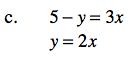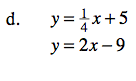### Home > CAAC > Chapter 6 > Lesson 6.3.1 > Problem6-99

6-99.
1. Find the point of intersection for each set of equations below using any method. Check your solutions, if possible. Homework Help ✎

1. 6x − 2y = 10
3x − 5 = y

2. 6x − 2y = 5
3x + 2y = −2

3. 5 − y = 3x
y = 2x

4. y =+ 5
y = 2x − 9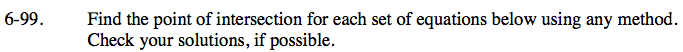For more help with this problem, see problem 6-71.

Substitution is one way to solve this system.

Distribute the left side.

Combine like terms.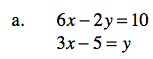6x − 2(3x − 5) = 10

6x − 6x + 10 = 10

10 = 10

All numbers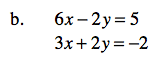Elimination is one method to solve this system.

$\left(\frac{1}{3},-\frac{3}{2}\right)$